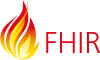# The range of the number value (precision of the value）

[parameter]=100 ， Values that equal 100, to 3 significant figures precision, so this is actually searching for values in the range [99.5 … 100.5)
and
[parameter]=100.00， Values that equal 100, to 5 significant figures precision, so this is actually searching for values in the range [99.995 … 100.005)

in the case , the 2.0 should:
[parameter]=2.0, Values that equal 2, to 2 significant figures precision, so this is actually searching for values in the range [1.5 … 2.5)
but the spec also said: The number 2.0 has a range of 1.95 to 2.05
I’m so confused.
what the “significant figures precision” used for? and how to seach Values that equal exactly 2.0 if the target value is decimals?

If you’re talking about something measured as a decimal, you’re talking about something that was measured - and measurements are never exact. They’re measured to some degree of precision. Something that you measure as 2.0 is in actually something that is 2.0251887… or 1.9723411…, etc. In the actual data, decimals can have a range of precisions. While one system might capture 2.0 for both of the previous numbers, another might capture 2.02 and 1.97 and another might capture 2.025 and 1.972. When you search by 2.0, you wouldn’t want 2.025 to be excluded because if someone had captured 2.025 with only 2 significant digits, it would have been 2.0 in the database. As a result, all decimal searches are always searches by range. (The same is true for dates.)

If you search for 2.0, it wouldn’t search in the range of 1.5 to 2.5, it would search in the range of 1.95 to 2.05. That’s the only way the 2.025 would be included in your search criteria. If you wanted to search for a decimal value that, when converted to a string would exactly match “2.0”, then you’d need to define a custom search criteria of type token that had that behavior - but it’s not clear why you’d actually want to do that…